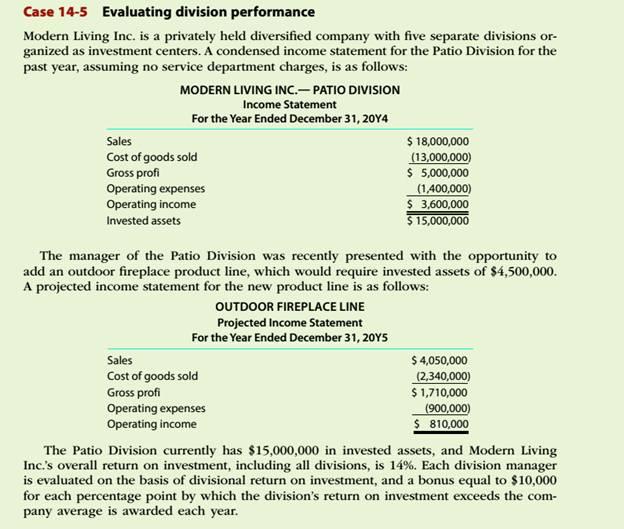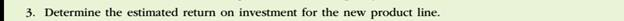Chapter
Section
Textbook ProblemTo determine

Concept Introduction:

Return on investment (ROI):

Return on investment is a profitability ratio that represents the percentage return on the investment made. It is calculated by dividing the Net Income by the Average total assets. The formulas to calculate the ROI are as follows:

ROI = Operating IncomeAverage total assets

ROI (Expanded)= Operating IncomeSales ×SalesAverage total assets

Or

ROI = Profit Margin Ratio ×Asset Turnover ratio

To Calculate:

The estimated return on investment for the new product line

Explanation

The estimated return on investment for the new product line is calculated as follows:

 Operating Income (A) \$ 810,000

Still sussing out bartleby?

Check out a sample textbook solution.

See a sample solution

The Solution to Your Study Problems

Bartleby provides explanations to thousands of textbook problems written by our experts, many with advanced degrees!

Get Started

Refer to an online finance source such as Yahoo! Finance or Google Finance to look up the P/E ratios for Verizo...

Fundamentals of Financial Management, Concise Edition (with Thomson ONE - Business School Edition, 1 term (6 months) Printed Access Card) (MindTap Course List)

How should the capital structure weights used to calculate the WACC be determined?

Fundamentals of Financial Management, Concise Edition (MindTap Course List)

Identify and describe the major types of teams.

Foundations of Business (MindTap Course List)

Briefly explain what is meant by the term efficiency continuum.

Fundamentals of Financial Management (MindTap Course List)